# Physical Chemistry : Physical Chemistry

## Example Questions

← Previous 1 3 4 5 6 7 8 9 17 18

### Example Question #1 : Thermochemistry And Thermodynamics

For Constant Temperature, Gibbs Free Energy is defined as: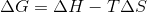Where ,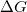is the change in Gibbs Free Energy,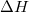is the change in enthalpy,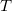is temperature, and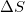is the change in entropy.

Which of the following scenarios is not possible?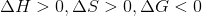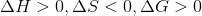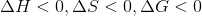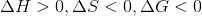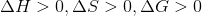Explanation:

The following condition is not possible:This is because if enthalpy is positive, and entropy is negative, the negative sign in front of the temperature term in the formula becomes positive. Addition of 2 positive numbers can not be negative. Plugging in arbitrary numbers into the other conditions can show they are all possible.

Take the following condition: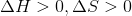Then Gibbs free energy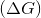can either be positive or negative, depending on the magnitude of enthalpy, entropy, and temperature. (If enthalpy is much larger than entropy and temperature, then the difference will be positive, but if entropy *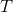is greater than the enthalpy, then the difference will be negative).

### Example Question #1 : Physical Chemistry

For constant temperature, Gibbs free energy is defined as:Where ,is the change in Gibbs free energy,is the change in enthalpy,is temperature, andis the change in entropy.

Given that a system is spontaneous, which of the following states are possible?

I.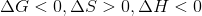II.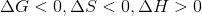III.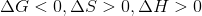IV.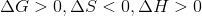I, II, III, and IV

I, II, and III

II and III

I and III

IV only

I and III

Explanation:

Condition I is always true. Condition II is never true, as Gibbs free energy cannot be negative if enthalpy is positive and entropy is negative. Condition III may be true if temperature is very high (this is the scenario when the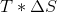term dominates the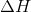term. Condition IV is not possible because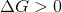and we were given a system with a Gibbs free Energy that is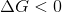(we were told the system was spontaneous).

### Example Question #1 : Gibbs Free Energy

The enthalpy of a reaction is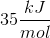and the entropy of a reaction is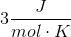. Which of the following is the Gibbs free energy (in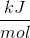) of this reaction?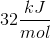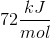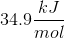Cannot be determined from the given information

Cannot be determined from the given information

Explanation:

Gibbs free energy of a system can be solved using the following equation.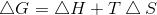where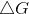is change in Gibbs free energy,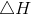is change in enthalpy,is temperature in Kelvins and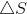is change in entropy. To solve forwe need all three of the variables. We are not given the temperature; therefore, we cannot solve for Gibbs free energy.

### Example Question #1 : Gibbs Free Energy

In an exergonic reaction, products will have __________ Gibbs free energy and the reaction is __________.

higher . . . spontaneous

higher . . . nonspontaneous

lower . . . nonspontaneous

lower . . . spontaneous

lower . . . spontaneous

Explanation:

Exergonic reaction suggests that the Gibbs free energy is negative. Since the change in Gibbs free energy is defined as Gibbs free energy of products - Gibbs free energy of reactants, a negative change in Gibbs free energy suggests that the products have a lower Gibbs free energy than reactants. A reaction is spontaneous if it has negative Gibbs free energy; therefore, exergonic reactions are always spontaneous. This is because the reaction is producing a more stable product (lower energy) from a less stable reactant (higher energy).

### Example Question #1 : Enthalpy And Entropy

Which of the following is true regarding enthalpy and entropy?

None of these are true

The entropy of liquid water is higher than the entropy of hydrogen gas

The enthalpy of liquid water is nonzero whereas enthalpy of hydrogen gas is zero

Both of these are true

The enthalpy of liquid water is nonzero whereas enthalpy of hydrogen gas is zero

Explanation:

Enthalpy is the amount of internal energy contained in a compound whereas entropy is the amount of intrinsic disorder within the compound. Enthalpy is zero for elemental compounds such hydrogen gas and oxygen gas; therefore, enthalpy is nonzero for water (regardless of phase). Entropy, or the amount of disorder, is always highest for gases and lowest for solids. This is because gas molecules are widely spread out and, therefore, are more disordered than solids and liquids. Hydrogen gas will have a higher entropy than liquid water.

### Example Question #1 : Enthalpy And Entropy

According to the law of thermodynamics, which of the following statement(s) is/are true?

I. Enthalpy of a system is always increasing

II. Entropy of a system is always increasing

III. Absolute entropy can never be negative

III only

II only

I and II

II and III

III only

Explanation:

The first law of thermodynamics states that the energy of the universe is always constant, which implies that energy cannot be created or destroyed. The energy lost by a system is gained by surroundings and vice versa; however, the total energy of the universe is always constant. The second law of thermodynamics states that the entropy, or the amount of disorder in the universe, is always increasing. This suggests that the universe is always going towards a more disordered state. Based on these two laws, we can determine that statement I and statement II are false. Note that these two statements are talking about the system, rather than the universe. The energy (in the form of enthalpy) and entropy can increase or decrease in a system. The surroundings will compensate accordingly to keep the energy of universe constant and increase the entropy. Absolute entropy of a system, surroundings or the universe can never be negative because it isn’t possible to have negative disorder (this is due to the definition of entropy; just remember that entropy can never be negative). Note that the change in entropy can, however, be negative.

### Example Question #1 : Laws Of Thermodynamics

A newly discovered element has been determined to have a standard entropy of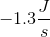in its pure form. This value is highly unlikely because it violates the __________.

Second Law of Thermodynamics

First Law of Equivalent Exchange

First Law of Thermodynamics

Third Law of Thermodynamics

Zeroth Law of Thermodynamics

Third Law of Thermodynamics

Explanation:

The Third Law of Thermodynamics states that a pure crystalline substance at absolute zero has an entropy of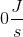. Mathematically, this formula is expressed as: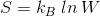where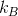is the Boltzmann constant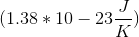and W is the number of microstates of a gaseous atom in the system. Because microstates are used to describe this gaseous atom, there has to be at least one or more.

If only one microstate exists for the system, then the entropy is zero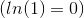, and can only increase from this point. It is therefore mathematically impossible for any element to have a negative standard entropy.

Remark: keep in mind that entropy changes can be negative, but the entropy of pure compounds cannot be negative.

### Example Question #1 : Thermochemistry And Thermodynamics

A chemical reaction is run in whichof work is done by the system and the internal energy changes by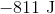. What is the total amount of heat transferred?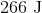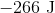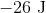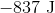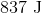Explanation:

The First Law of thermodynamics states that for a system that only exchanges energy by heat or work: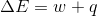Work is done by the system, therefore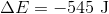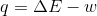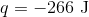### Example Question #1 : Heat Transfer

An automobile engine provides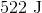of work to push the pistons and generates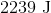of heat that must be carried away by the cooling system. What is the change in the internal energy of the engine?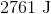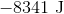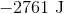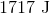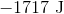Explanation:

The First Law of thermodynamics states that for a system that only exchanges energy by heat or work:The heat is given off by the system, so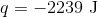. Similarly, work is being done by the system, so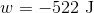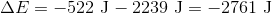### Example Question #1 : Physical Chemistry

A chemical reaction is run in which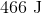of heat is absorbed and the internal energy changes by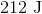. What is the amount of work done?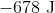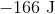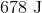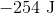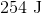Explanation:

The First Law of thermodynamics states that for a system that only exchanges energy by heat or work:Heat is absorbed by the system, therefore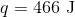So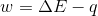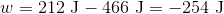done by the system.

← Previous 1 3 4 5 6 7 8 9 17 18

### All Physical Chemistry Resources